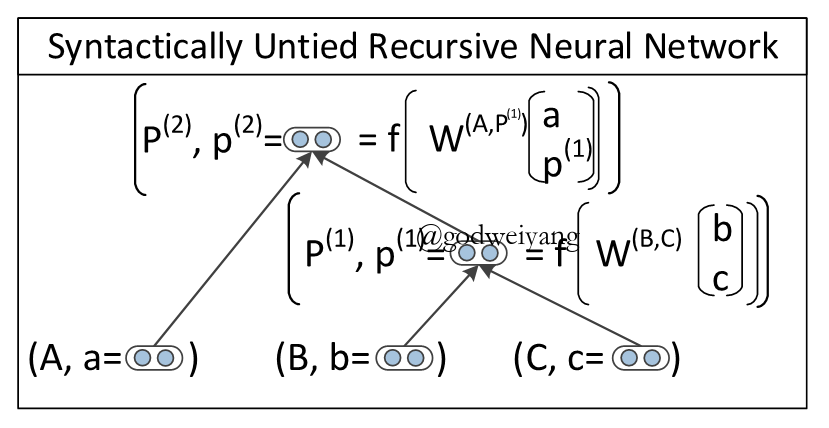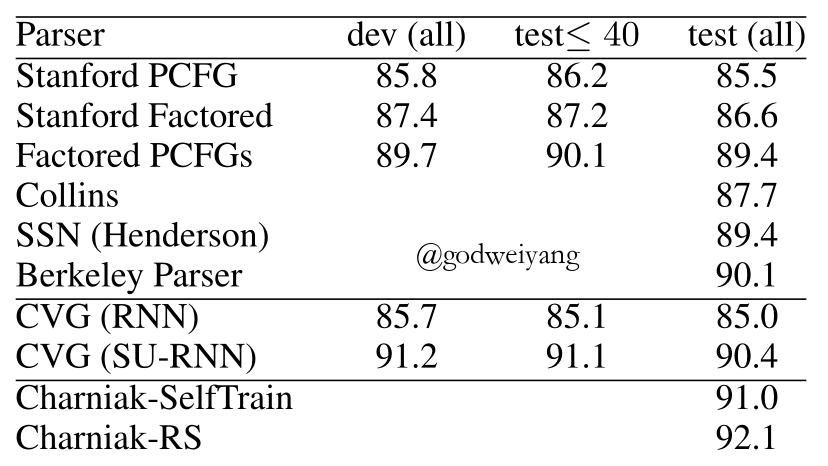Parsing with Compositional Vector Grammars# 模型${p^{(1)}} = f\left( { {W^{(B,C)}}\left[ {\begin{array}{*{20}{c}}b\\c\end{array}} \right]} \right)$而这个节点的得分表示为
$s({p^{(1)}}) = {({v^{(B,C)}})^T}{p^{(1)}} + \log P({P_1} \to B{\rm{ }}C)$最后一整棵树的得分就是
$s(CVG(\theta ,x,\hat y)) = \sum\limits_{d \in N(\hat y)} {s({p^d})}$这样就可以枚举所有的句法树，然后计算得到得分最高的那棵树就是最终的句法树了。

$\Delta ({y_i},\hat y) = \sum\limits_{d \in N(\hat y)} {\kappa 1\{ d \notin N({y_i})\} }$最终的损失函数定义为：
$J(\theta ) = \frac{1}{m}\sum\limits_{i = 1}^m { {r_i}(\theta )} + \frac{\lambda }{2}{\left| \theta \right|^2}$其中
${r_i}(\theta ) = \mathop {\max }\limits_{\hat y \in Y({x_i})} (s(CVG({x_i},\hat y)) + \Delta ({y_i},\hat y)) - s(CVG({x_i},{y_i}))$也就是要尽量最大化标准树的得分，减小预测树的得分。

# 实验结果# 我的毕设任务

转载规则

《Parsing with Compositional Vector Grammars》 韦阳 采用 知识共享署名 4.0 国际许可协议 进行许可。
上一篇Head-Lexicalized Bidirectional Tree LSTMs

2018-01-13Empower Sequence Labeling with Task-Aware Neural Language Model

2018-01-09
目录